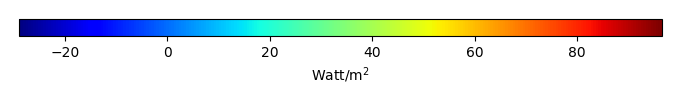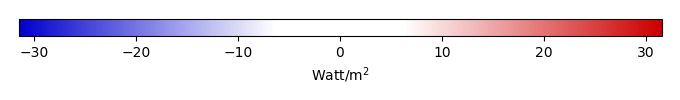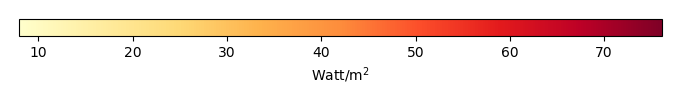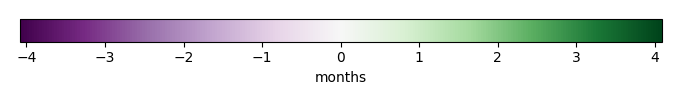# Mean State

Period Mean (original grids) [Watt/m2]
Model Period Mean (intersection) [Watt/m2]
Model Period Mean (complement) [Watt/m2]
Benchmark Period Mean (intersection) [Watt/m2]
Benchmark Period Mean (complement) [Watt/m2]
Bias [Watt/m2]
RMSE [Watt/m2]
Phase Shift [months]
Bias Score 
RMSE Score 
Seasonal Cycle Score 
Spatial Distribution Score 
Overall Score 
Benchmark [-] 44.1
CLM4 [-] 44.9 44.2 57.8 44.2 12.4 0.430 24.4 1.42 0.333 0.154 0.789 0.694 0.425
CLM4.5 [-] 52.4 51.5 63.6 44.2 12.4 7.70 18.2 1.48 0.423 0.221 0.777 0.830 0.495
CLM5 [-] 51.8 51.1 64.4 44.2 12.4 7.31 18.6 1.46 0.414 0.241 0.789 0.761 0.489
Period Mean (original grids) [Watt/m2]
Model Period Mean (intersection) [Watt/m2]
Model Period Mean (complement) [Watt/m2]
Benchmark Period Mean (intersection) [Watt/m2]
Benchmark Period Mean (complement) [Watt/m2]
Bias [Watt/m2]
RMSE [Watt/m2]
Phase Shift [months]
Bias Score 
RMSE Score 
Seasonal Cycle Score 
Spatial Distribution Score 
Overall Score 
Benchmark [-] 57.5
CLM4 [-] 55.9 56.0 84.3 57.5 20.8 -1.35 20.8 0.916 0.527 0.380 0.899 0.959 0.629
CLM4.5 [-] 56.8 57.0 89.4 57.5 20.8 -0.402 20.4 1.05 0.545 0.377 0.884 0.968 0.630
CLM5 [-] 59.0 59.1 89.7 57.5 20.8 1.68 21.6 1.33 0.533 0.362 0.846 0.970 0.615
Period Mean (original grids) [Watt/m2]
Model Period Mean (intersection) [Watt/m2]
Model Period Mean (complement) [Watt/m2]
Benchmark Period Mean (intersection) [Watt/m2]
Benchmark Period Mean (complement) [Watt/m2]
Bias [Watt/m2]
RMSE [Watt/m2]
Phase Shift [months]
Bias Score 
RMSE Score 
Seasonal Cycle Score 
Spatial Distribution Score 
Overall Score 
Benchmark [-] 52.8
CLM4 [-] 56.0 55.8 62.2 53.0 19.6 3.12 19.8 0.619 0.581 0.414 0.943 0.947 0.660
CLM4.5 [-] 56.7 56.5 64.3 53.0 19.6 3.86 18.4 0.632 0.607 0.445 0.942 0.969 0.681
CLM5 [-] 58.2 57.9 66.6 53.0 19.6 5.27 19.2 0.627 0.556 0.465 0.942 0.935 0.673
Period Mean (original grids) [Watt/m2]
Model Period Mean (intersection) [Watt/m2]
Model Period Mean (complement) [Watt/m2]
Benchmark Period Mean (intersection) [Watt/m2]
Benchmark Period Mean (complement) [Watt/m2]
Bias [Watt/m2]
RMSE [Watt/m2]
Phase Shift [months]
Bias Score 
RMSE Score 
Seasonal Cycle Score 
Spatial Distribution Score 
Overall Score 
Benchmark [-] 18.0
CLM4 [-] 3.35 3.62 -0.834 18.1 5.64 -14.7 25.0 0.781 0.545 0.464 0.940 0.798 0.642
CLM4.5 [-] 3.40 3.67 -1.25 18.1 5.64 -14.8 23.0 0.691 0.545 0.509 0.950 0.797 0.662
CLM5 [-] -0.719 -0.431 -5.58 18.1 5.64 -18.8 25.0 0.610 0.465 0.552 0.949 0.525 0.609
Period Mean (original grids) [Watt/m2]
Model Period Mean (intersection) [Watt/m2]
Model Period Mean (complement) [Watt/m2]
Benchmark Period Mean (intersection) [Watt/m2]
Benchmark Period Mean (complement) [Watt/m2]
Bias [Watt/m2]
RMSE [Watt/m2]
Phase Shift [months]
Bias Score 
RMSE Score 
Seasonal Cycle Score 
Spatial Distribution Score 
Overall Score 
Benchmark [-] 22.1
CLM4 [-] 20.7 19.1 28.6 22.8 10.8 -0.765 19.8 1.56 0.236 0.135 0.775 0.457 0.347
CLM4.5 [-] 40.4 39.5 44.6 22.8 10.8 18.5 25.5 1.41 0.126 0.120 0.804 0.661 0.366
CLM5 [-] 29.9 28.6 36.4 22.8 10.8 8.44 22.3 1.78 0.135 0.144 0.730 0.415 0.314
Period Mean (original grids) [Watt/m2]
Model Period Mean (intersection) [Watt/m2]
Model Period Mean (complement) [Watt/m2]
Benchmark Period Mean (intersection) [Watt/m2]
Benchmark Period Mean (complement) [Watt/m2]
Bias [Watt/m2]
RMSE [Watt/m2]
Phase Shift [months]
Bias Score 
RMSE Score 
Seasonal Cycle Score 
Spatial Distribution Score 
Overall Score 
Benchmark [-] 27.0
CLM4 [-] 21.8 21.9 17.1 27.1 8.81 -5.09 16.9 0.497 0.759 0.571 0.962 0.970 0.767
CLM4.5 [-] 17.0 17.1 18.0 27.1 8.81 -9.91 19.0 0.982 0.652 0.570 0.904 0.947 0.729
CLM5 [-] 10.9 10.9 15.3 27.1 8.81 -16.1 22.4 0.994 0.536 0.579 0.899 0.878 0.694
Period Mean (original grids) [Watt/m2]
Model Period Mean (intersection) [Watt/m2]
Model Period Mean (complement) [Watt/m2]
Benchmark Period Mean (intersection) [Watt/m2]
Benchmark Period Mean (complement) [Watt/m2]
Bias [Watt/m2]
RMSE [Watt/m2]
Phase Shift [months]
Bias Score 
RMSE Score 
Seasonal Cycle Score 
Spatial Distribution Score 
Overall Score 
Benchmark [-] 28.4
CLM4 [-] 36.3 36.0 39.4 28.4 24.4 7.48 20.3 0.981 0.665 0.467 0.882 0.907 0.678
CLM4.5 [-] 35.0 34.5 40.5 28.4 24.4 5.98 21.0 1.01 0.662 0.450 0.878 0.903 0.669
CLM5 [-] 35.2 34.7 40.6 28.4 24.4 6.23 20.7 1.05 0.669 0.468 0.871 0.920 0.679
Period Mean (original grids) [Watt/m2]
Model Period Mean (intersection) [Watt/m2]
Model Period Mean (complement) [Watt/m2]
Benchmark Period Mean (intersection) [Watt/m2]
Benchmark Period Mean (complement) [Watt/m2]
Bias [Watt/m2]
RMSE [Watt/m2]
Phase Shift [months]
Bias Score 
RMSE Score 
Seasonal Cycle Score 
Spatial Distribution Score 
Overall Score 
Benchmark [-] 40.3
CLM4 [-] 48.8 48.8 51.1 40.6 11.0 7.34 23.6 0.524 0.555 0.382 0.960 0.898 0.635
CLM4.5 [-] 53.3 53.3 56.9 40.6 11.0 12.1 24.2 0.528 0.523 0.396 0.957 0.954 0.645
CLM5 [-] 52.0 52.0 56.2 40.6 11.0 10.9 23.0 0.686 0.541 0.425 0.938 0.939 0.653
Period Mean (original grids) [Watt/m2]
Model Period Mean (intersection) [Watt/m2]
Model Period Mean (complement) [Watt/m2]
Benchmark Period Mean (intersection) [Watt/m2]
Benchmark Period Mean (complement) [Watt/m2]
Bias [Watt/m2]
RMSE [Watt/m2]
Phase Shift [months]
Bias Score 
RMSE Score 
Seasonal Cycle Score 
Spatial Distribution Score 
Overall Score 
Benchmark [-] 15.2
CLM4 [-] 0.805 1.30 -7.30 15.2 3.78 -14.2 24.5 0.934 0.568 0.464 0.930 0.673 0.620
CLM4.5 [-] -2.17 -1.72 -9.51 15.2 3.78 -17.2 24.2 1.01 0.499 0.517 0.913 0.462 0.582
CLM5 [-] -9.16 -8.77 -15.8 15.2 3.78 -24.2 28.4 0.787 0.376 0.568 0.942 0.203 0.531
Period Mean (original grids) [Watt/m2]
Model Period Mean (intersection) [Watt/m2]
Model Period Mean (complement) [Watt/m2]
Benchmark Period Mean (intersection) [Watt/m2]
Benchmark Period Mean (complement) [Watt/m2]
Bias [Watt/m2]
RMSE [Watt/m2]
Phase Shift [months]
Bias Score 
RMSE Score 
Seasonal Cycle Score 
Spatial Distribution Score 
Overall Score 
Benchmark [-] 56.5
CLM4 [-] 63.6 64.2 51.3 56.9 15.0 6.74 19.7 0.712 0.708 0.498 0.940 0.970 0.723
CLM4.5 [-] 63.9 64.3 54.1 56.9 15.0 7.00 20.4 0.717 0.706 0.488 0.939 0.945 0.713
CLM5 [-] 64.1 64.8 51.4 56.9 15.0 7.25 18.2 0.602 0.700 0.560 0.954 0.985 0.752
Period Mean (original grids) [Watt/m2]
Model Period Mean (intersection) [Watt/m2]
Model Period Mean (complement) [Watt/m2]
Benchmark Period Mean (intersection) [Watt/m2]
Benchmark Period Mean (complement) [Watt/m2]
Bias [Watt/m2]
RMSE [Watt/m2]
Phase Shift [months]
Bias Score 
RMSE Score 
Seasonal Cycle Score 
Spatial Distribution Score 
Overall Score 
Benchmark [-] 20.2
CLM4 [-] 27.3 27.2 29.5 20.4 7.08 7.03 21.4 0.578 0.665 0.481 0.935 0.740 0.661
CLM4.5 [-] 26.5 26.4 29.4 20.4 7.08 6.26 21.8 0.506 0.667 0.472 0.942 0.703 0.651
CLM5 [-] 21.2 21.0 25.6 20.4 7.08 1.11 22.2 0.696 0.680 0.472 0.917 0.581 0.624
Period Mean (original grids) [Watt/m2]
Model Period Mean (intersection) [Watt/m2]
Model Period Mean (complement) [Watt/m2]
Benchmark Period Mean (intersection) [Watt/m2]
Benchmark Period Mean (complement) [Watt/m2]
Bias [Watt/m2]
RMSE [Watt/m2]
Phase Shift [months]
Bias Score 
RMSE Score 
Seasonal Cycle Score 
Spatial Distribution Score 
Overall Score 
Benchmark [-] 34.3
CLM4 [-] 33.0 38.0 12.6 34.6 11.5 3.29 21.9 0.886 0.586 0.429 0.909 0.874 0.645
CLM4.5 [-] 34.3 39.3 13.9 34.6 11.5 4.63 22.3 0.954 0.574 0.434 0.901 0.856 0.640
CLM5 [-] 32.2 37.2 11.2 34.6 11.5 2.60 22.5 1.02 0.556 0.456 0.894 0.788 0.630
Period Mean (original grids) [Watt/m2]
Model Period Mean (intersection) [Watt/m2]
Model Period Mean (complement) [Watt/m2]
Benchmark Period Mean (intersection) [Watt/m2]
Benchmark Period Mean (complement) [Watt/m2]
Bias [Watt/m2]
RMSE [Watt/m2]
Phase Shift [months]
Bias Score 
RMSE Score 
Seasonal Cycle Score 
Spatial Distribution Score 
Overall Score 
Benchmark [-] 22.7
CLM4 [-] 55.9 56.9 55.0 22.8 18.3 34.8 43.4 1.16 0.499 0.457 0.947 0.789 0.630
CLM4.5 [-] 56.4 57.5 55.3 22.8 18.3 35.5 44.2 1.12 0.496 0.444 0.953 0.794 0.626
CLM5 [-] 56.4 58.3 54.6 22.8 18.3 36.2 44.1 1.34 0.511 0.449 0.931 0.798 0.627
Period Mean (original grids) [Watt/m2]
Model Period Mean (intersection) [Watt/m2]
Model Period Mean (complement) [Watt/m2]
Benchmark Period Mean (intersection) [Watt/m2]
Benchmark Period Mean (complement) [Watt/m2]
Bias [Watt/m2]
RMSE [Watt/m2]
Phase Shift [months]
Bias Score 
RMSE Score 
Seasonal Cycle Score 
Spatial Distribution Score 
Overall Score 
Benchmark [-] 17.0
CLM4 [-] 6.67 7.42 -5.67 17.0 4.51 -10.3 21.2 0.638 0.679 0.531 0.959 0.751 0.690
CLM4.5 [-] 4.51 5.11 -5.87 17.0 4.51 -12.4 21.4 0.814 0.624 0.550 0.932 0.708 0.673
CLM5 [-] -1.36 -0.796 -10.9 17.0 4.51 -18.3 25.6 1.08 0.501 0.545 0.890 0.488 0.594
Period Mean (original grids) [Watt/m2]
Model Period Mean (intersection) [Watt/m2]
Model Period Mean (complement) [Watt/m2]
Benchmark Period Mean (intersection) [Watt/m2]
Benchmark Period Mean (complement) [Watt/m2]
Bias [Watt/m2]
RMSE [Watt/m2]
Phase Shift [months]
Bias Score 
RMSE Score 
Seasonal Cycle Score 
Spatial Distribution Score 
Overall Score 
Benchmark [-] 47.7
CLM4 [-] 53.2 52.6 59.9 48.2 15.9 4.69 25.1 0.534 0.461 0.501 0.960 0.689 0.622
CLM4.5 [-] 54.0 53.2 62.3 48.2 15.9 5.51 26.8 0.493 0.449 0.481 0.962 0.669 0.608
CLM5 [-] 54.1 53.3 62.2 48.2 15.9 5.59 24.9 0.650 0.463 0.520 0.945 0.681 0.626
Period Mean (original grids) [Watt/m2]
Model Period Mean (intersection) [Watt/m2]
Model Period Mean (complement) [Watt/m2]
Benchmark Period Mean (intersection) [Watt/m2]
Benchmark Period Mean (complement) [Watt/m2]
Bias [Watt/m2]
RMSE [Watt/m2]
Phase Shift [months]
Bias Score 
RMSE Score 
Seasonal Cycle Score 
Spatial Distribution Score 
Overall Score 
Benchmark [-] 28.6
CLM4 [-] 32.1 32.3 28.0 28.7 12.1 3.73 15.8 1.42 0.694 0.427 0.789 0.916 0.650
CLM4.5 [-] 31.3 31.4 31.4 28.7 12.1 2.84 17.6 2.19 0.678 0.376 0.666 0.928 0.605
CLM5 [-] 31.5 31.5 33.6 28.7 12.1 3.05 17.9 1.89 0.629 0.401 0.702 0.826 0.592
Period Mean (original grids) [Watt/m2]
Model Period Mean (intersection) [Watt/m2]
Model Period Mean (complement) [Watt/m2]
Benchmark Period Mean (intersection) [Watt/m2]
Benchmark Period Mean (complement) [Watt/m2]
Bias [Watt/m2]
RMSE [Watt/m2]
Phase Shift [months]
Bias Score 
RMSE Score 
Seasonal Cycle Score 
Spatial Distribution Score 
Overall Score 
Benchmark [-] 26.7
CLM4 [-] 29.9 30.2 17.3 26.9 8.98 2.89 17.1 0.511 0.773 0.582 0.958 0.925 0.764
CLM4.5 [-] 25.3 25.4 20.2 26.9 8.98 -1.72 15.4 0.686 0.784 0.627 0.939 0.974 0.790
CLM5 [-] 17.4 17.4 17.9 26.9 8.98 -9.57 19.3 1.01 0.660 0.603 0.892 0.951 0.742
Period Mean (original grids) [Watt/m2]
Model Period Mean (intersection) [Watt/m2]
Model Period Mean (complement) [Watt/m2]
Benchmark Period Mean (intersection) [Watt/m2]
Benchmark Period Mean (complement) [Watt/m2]
Bias [Watt/m2]
RMSE [Watt/m2]
Phase Shift [months]
Bias Score 
RMSE Score 
Seasonal Cycle Score 
Spatial Distribution Score 
Overall Score 
Benchmark [-] 49.7
CLM4 [-] 38.9 38.9 60.0 49.8 11.0 -10.8 19.0 0.577 0.641 0.657 0.955 0.890 0.760
CLM4.5 [-] 37.0 37.0 64.1 49.8 11.0 -12.7 21.3 0.587 0.595 0.638 0.955 0.933 0.752
CLM5 [-] 37.7 37.8 60.2 49.8 11.0 -11.9 19.2 0.577 0.636 0.657 0.950 0.918 0.764
Period Mean (original grids) [Watt/m2]
Model Period Mean (intersection) [Watt/m2]
Model Period Mean (complement) [Watt/m2]
Benchmark Period Mean (intersection) [Watt/m2]
Benchmark Period Mean (complement) [Watt/m2]
Bias [Watt/m2]
RMSE [Watt/m2]
Phase Shift [months]
Bias Score 
RMSE Score 
Seasonal Cycle Score 
Spatial Distribution Score 
Overall Score 
Benchmark [-] 30.2
CLM4 [-] 26.9 26.7 48.0 30.3 13.0 -2.93 17.7 1.52 0.378 0.165 0.801 0.484 0.399
CLM4.5 [-] 44.3 44.3 53.8 30.3 13.0 14.3 20.5 1.33 0.240 0.175 0.834 0.834 0.452
CLM5 [-] 36.5 36.4 50.4 30.3 13.0 6.57 16.1 1.45 0.329 0.191 0.813 0.701 0.445
Period Mean (original grids) [Watt/m2]
Model Period Mean (intersection) [Watt/m2]
Model Period Mean (complement) [Watt/m2]
Benchmark Period Mean (intersection) [Watt/m2]
Benchmark Period Mean (complement) [Watt/m2]
Bias [Watt/m2]
RMSE [Watt/m2]
Phase Shift [months]
Bias Score 
RMSE Score 
Seasonal Cycle Score 
Spatial Distribution Score 
Overall Score 
Benchmark [-] 40.3
CLM4 [-] 43.7 43.8 44.0 40.4 9.36 3.57 17.7 1.12 0.545 0.357 0.883 0.799 0.588
CLM4.5 [-] 46.4 46.5 45.9 40.4 9.36 6.26 16.7 1.15 0.579 0.374 0.877 0.893 0.619
CLM5 [-] 46.8 47.0 43.6 40.4 9.36 6.69 16.7 0.967 0.515 0.422 0.905 0.778 0.608
Period Mean (original grids) [Watt/m2]
Model Period Mean (intersection) [Watt/m2]
Model Period Mean (complement) [Watt/m2]
Benchmark Period Mean (intersection) [Watt/m2]
Benchmark Period Mean (complement) [Watt/m2]
Bias [Watt/m2]
RMSE [Watt/m2]
Phase Shift [months]
Bias Score 
RMSE Score 
Seasonal Cycle Score 
Spatial Distribution Score 
Overall Score 
Benchmark [-] 41.5
CLM4 [-] 57.4 56.9 61.6 42.0 6.56 13.7 28.1 0.839 0.607 0.501 0.908 0.936 0.691
CLM4.5 [-] 56.1 55.7 61.5 42.0 6.56 12.9 27.8 0.823 0.609 0.508 0.910 0.934 0.694
CLM5 [-] 56.9 56.4 61.4 42.0 6.56 13.2 26.8 0.781 0.610 0.549 0.930 0.938 0.715

# Temporally integrated period mean

BENCHMARK MEANMODEL MEANBIASBIAS SCORERMSERMSE SCOREBENCHMARK MAX MONTHMODEL MAX MONTHDIFFERENCE IN MAX MONTHSEASONAL CYCLE SCORESPATIAL TAYLOR DIAGRAMMODEL COLORS# Spatially integrated regional mean

MODEL COLORSREGIONAL MEANANNUAL CYCLEMONTHLY ANOMALYANNUAL CYCLE# All Models

BenchmarkCLM4CLM4.5CLM5# Data Information

creation_date: Tue Jul 28 15:54:11 PDT 2015

source_file: This product is generated from monthly Global Biosphere Amtospher Flux (GBAF) observations

title: derived GBAF in 0.5x0.5

Approach: I read this variable from data file, and then save the data in NetCDF format by each month and each year. Meanwhile, I also converted the unit from MJoule*m-2*d-1 to Watt/m2.

Temporal resolution: monthly

General information: This product was derived from Monthly estimates of global biosphere-atmosphere fluxes from Biogeochemistry group at Max Planck Institute in Jena, Germany.

Spatial resolution: 0.5x0.5 degree